# High School Physics : Impulse and Momentum

## Example Questions

### Example Question #61 : Momentum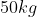crate slides along the floor for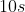before stopping. If it was initially moving with a velocity of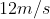, what is the force of friction?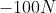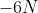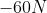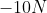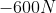Explanation:

The fastest way to solve a problem like this is with momentum.

Remember that momentum is equal to mass times velocity: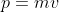. We can rewrite this equation in terms of force.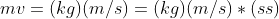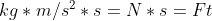Using this transformation, we can see that momentum is also equal to force times time.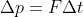can also be thought of as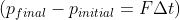.

Expand this equation to include our given values.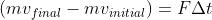Since the object is not moving at the end, its final velocity is zero. Plug in the given values and solve for the force.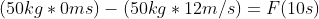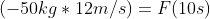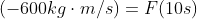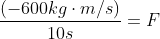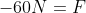We would expect the answer to be negative because the force of friction acts in the direction opposite to the initial velocity.

### Example Question #62 : Momentum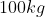crate slides along a floor with a starting velocity of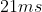. If the force due to friction is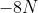, how long will it take for the box to come to rest?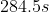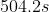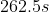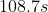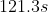Explanation:

The fastest way to solve a problem like this is with momentum.

Remember that momentum is equal to mass times velocity:. We can rewrite this equation in terms of force.Using this transformation, we can see that momentum is also equal to force times time.can also be thought of as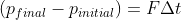.

Expand this equation to include our given values.Since the box is not moving at the end, its final velocity is zero. Plug in the given values and solve for the time.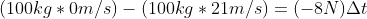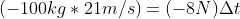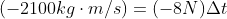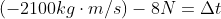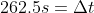### Example Question #1 : Impulse And Momentum

A man with a mass of m is painting a house. He stands on a tall ladder of height h. He leans over and falls straight down off the ladder. If he is in the air for s seconds, what will be his momentum right before he hits the ground?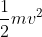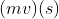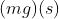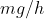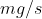Explanation:

The problem tells us he falls vertically off the ladder (straight down), so we don't need to worry about motion in the horizontal direction.

The equation for momentum is:We can assume he falls from rest, which allows us to find the initial momentum.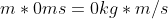.

From here, we can use the formula for impulse: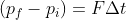We know his initial momentum is zero, so we can remove this variable from the equation.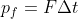The problem tells us that his change in time is s seconds, so we can insert this in place of the time.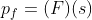The only force acting upon man is the force due to gravity, which will always be given by the equation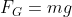.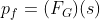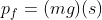### Example Question #64 : Momentum

If an egg is dropped on concrete, it usually breaks. If an egg is dropped on grass, it may not break. What conclusion explains this result?

The egg is in contact with the grass for longer, so it absorbs less force

Grass has less mass than concrete

The coefficient of friction of the grass on the egg is less than the coefficient of the concrete on the egg

Grass is a softer texture

The density of grass is less than the density of concrete

The egg is in contact with the grass for longer, so it absorbs less force

Explanation:

In both cases the egg starts with the same velocity, so it has the same initial momentum. In both cases the egg stops moving at the end of its fall, so it has the same final velocity. The only thing that changes is the time and force of the impact. The force is produced by the deceleration resulting from the time that the egg is in contact with its point of impact.

As the time of contact increases, acceleration decreases and force decreases.

As the time of contact decreases, acceleration increases and force increases.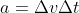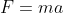The grass will have less force on the egg, allowing for a lesser acceleration, due to a longer period of impact.

### Example Question #65 : Momentum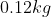tennis ball strikes a racket, moving at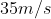. After striking the racket, it bounces back at a speed of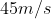. What is the change in momentum?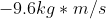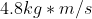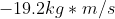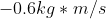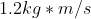Explanation:

The change in momentum is the final momentum minus the initial momentum, or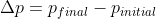.

Notice that the problem gives us the final SPEED of the ball but not the final VELOCITY. Since the ball "bounced back," it begins to move in the opposite direction, so its velocity at this point will be negative.

Plug in our values to solve: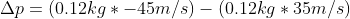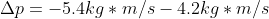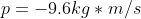### Example Question #1 : Impulse And Momentum

The area under the curve on a Force versus time (F versus t) graph represents

Work

Momentum

Kinetic energy

Impulse